# splines

Controls how, and if, edges are represented

type: bool | string

If `splines=true`, edges are drawn as splines routed around nodes; if `splines=false`, edges are drawn as line segments. If `splines=none` or `splines=""`, no edges are drawn at all.

(1 March 2007) `splines=line` and `splines=spline` can be used as synonyms for `splines=false` and `splines=true`, respectively.

In addition, `splines=polyline` specifies that edges should be drawn as polylines.

(28 Sep 2010) `splines=ortho` specifies edges should be routed as polylines of axis-aligned segments. Currently, the routing does not handle ports or, in dot, edge labels.

(25 Sep 2012) `splines=curved` specifies edges should be drawn as curved arcs.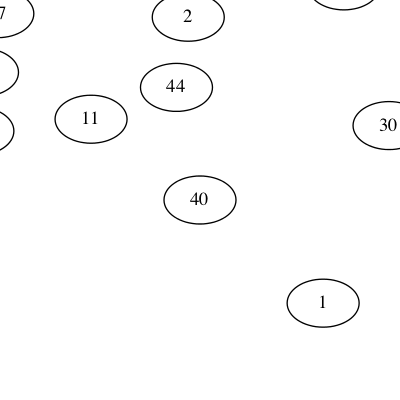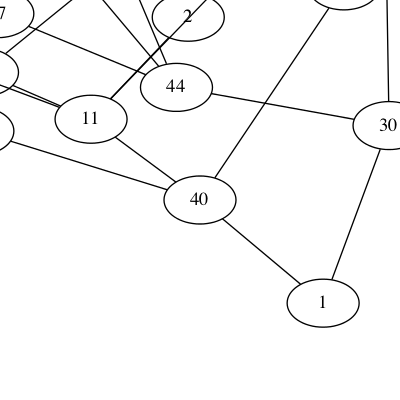splines=nonesplines="" splines=linesplines=false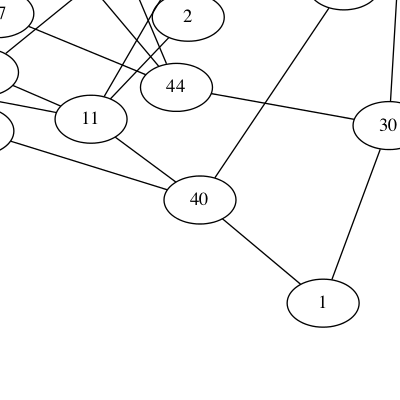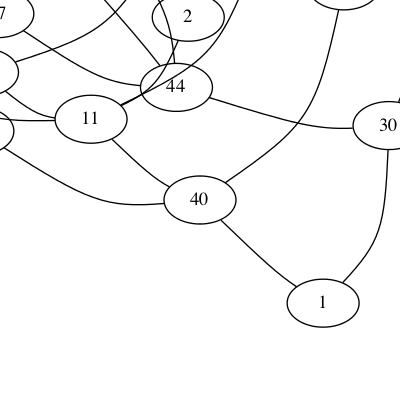splines=polyline splines=curved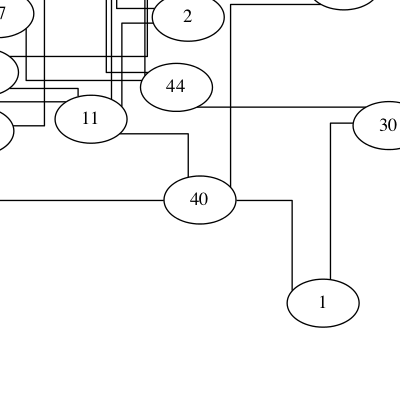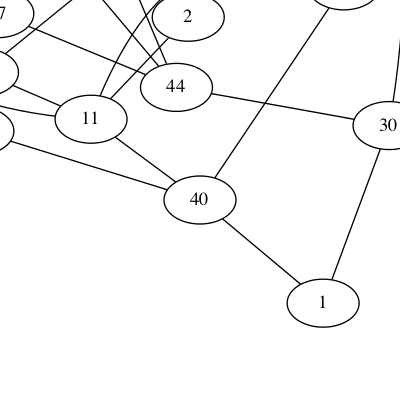splines=ortho splines=splinesplines=true

By default, `splines` is unset. How this is interpreted depends on the layout engine. For `dot`, the default is to draw edges as splines. For all other layouts, the default is to draw edges as line segments.

Note that for these latter layouts, if `splines="true"`, this requires non-overlapping nodes (cf. `overlap`). If `fdp` is used for layout and `splines="compound"`, then the edges are drawn to avoid clusters as well as nodes.

Valid on:
• Graphs